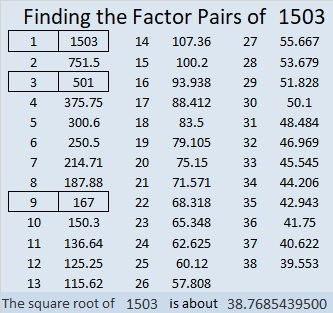# Why is 1503 a Friedman Number?

Contents

### Friedman Puzzle:

Can you find an expression equaling 1503 that uses 1, 5, 0, and 3 each exactly once, but in any order, and some combination of  +, -, ×, or ÷? For this particular Friedman puzzle, none of those digits are exponents. If you can solve this Friedman puzzle, you will know why 1503 is the 24th Friedman number. You can find the solution hidden someplace in this post. (By the way, another permutation of those digits, 1530, will be the 25th Friedman number!)

### Find the Factors Puzzle:

There are 14 clues in this level 2 puzzle. Use those clues and logic to place the factors 1 to 10 in both the first column and the top row. That’s how you start to turn this puzzle into a multiplication table!### Factors of 1503:

• 1503 is a composite number.
• Prime factorization: 1503 = 3 × 3 × 167, which can be written 1503 = 3² × 167.
• 1503 has at least one exponent greater than 1 in its prime factorization so √1503 can be simplified. Taking the factor pair from the factor pair table below with the largest square number factor, we get √1503 = (√9)(√167) = 3√167.
• The exponents in the prime factorization are 2 and 1. Adding one to each exponent and multiplying we get (2 + 1)(1 + 1) = 3 × 2 = 6. Therefore 1503 has exactly 6 factors.
• The factors of 1503 are outlined with their factor pair partners in the graphic below.Did you see the solution to the Friedman puzzle in that factor pair chart?

This site uses Akismet to reduce spam. Learn how your comment data is processed.